# Test: Matrices & Determinants (Competition Level)

## 30 Questions MCQ Test Mathematics (Maths) Class 12 | Test: Matrices & Determinants (Competition Level)

Description
This mock test of Test: Matrices & Determinants (Competition Level) for JEE helps you for every JEE entrance exam. This contains 30 Multiple Choice Questions for JEE Test: Matrices & Determinants (Competition Level) (mcq) to study with solutions a complete question bank. The solved questions answers in this Test: Matrices & Determinants (Competition Level) quiz give you a good mix of easy questions and tough questions. JEE students definitely take this Test: Matrices & Determinants (Competition Level) exercise for a better result in the exam. You can find other Test: Matrices & Determinants (Competition Level) extra questions, long questions & short questions for JEE on EduRev as well by searching above.
QUESTION: 1

### The number of different orders of a matrix having 12 elements is

Solution:

(1,12),(2,6),(3,4(,(4,3),(6,2),(12,1)
6 different matrices.

QUESTION: 2

###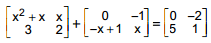then x is equal to

Solution:

x²+x=0
x(x+1)=0
therefore x=0 or -1
3-x+1=5
x=-1.
Hence, x = -1

QUESTION: 3

### If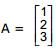and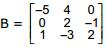, then

Solution:

No, we cannot multiply 3*1 by 3*3, So it doesnt exist.

QUESTION: 4

If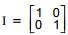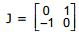and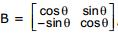, then B equal to

Solution:
QUESTION: 5

If A and B are square matrices of order 2, then (A + B)2 equal to

Solution:

(A+B)2 = (A+B) (A+B)
= A(A+B) +B(A+B)
A2 + AB + BA + B2

QUESTION: 6

If A is a skew – symmetric matrix, then trace of A is equal to

Solution:

We know that for a skew-symmetric matrix the sum of diagonal elements is zero.
a[ij] = 0∀i = j
So,tr(A) = 0

QUESTION: 7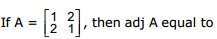Solution:
QUESTION: 8

Which of the following is a vector?

Solution:

Acceleration is a vector quantity that is defined as the rate at which an object changes its velocity. An object is accelerating if it is changing its velocity.

QUESTION: 9

If A is a square matrix such that A2  =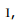then A–1 equal to

Solution:

As we know that, A2 = I
On multiplying both sides by A-1
(A2)A-1 = I A-1
A = I A-1
A = A-1

QUESTION: 10

If A and B are square matrices of order 3 such that |A| = –1, |B| = 3, then |3AB| is equal to

Solution:

∣AB∣=∣A∣∣B∣
Also for a square matrix of order 3
∣KA∣=K3∣A∣ because each element of the matrix A is multiplied by k and hence in this case we will have k3 common.
∴∣3AB∣ = (3)3
∣A∣∣B∣ = 27(−1)(3)
= −81

QUESTION: 11

If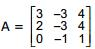, then value of A-1 is equal to

Solution:
QUESTION: 12

If the order of matrix A is m×p. And the order of B is p×n. Then the order of matrix AB is?

Solution:

If we are given 2 matrices of with order a×b and c×d, in order to be multiplicable, b must be equal to c and the resultant matrix will have order a×d. So using this formula in this question, We have p = p and so the resultant matrix will have m×n order. So, order of matrix AB is m×n.

QUESTION: 13

The system of equation –2x + y + z = 1, x – 2y + z = –2, x + y + λz = 4 will have no solution if

Solution:

D = 0
{(-2,1,1) (1,-2,1) ( 1,1, λ) = 0
= (4λ +1 +1) - (-2 - 2 + λ) = 0
4λ + 2 + 4 - λ = 0
3λ + 6 = 0
λ = -2

QUESTION: 14

The system of the linear equations x + y – z = 6, x + 2y – 3z = 14 and 2x + 5y – λz = 9 (λ ∈ R) has a unique solution if

Solution:

For a system of linear equations having unique solution, value of the determinant should not be 0
⇒ {(1,1,-1) (1,2,-3) (2,5,-λ)} not equal to o
⇒ [−2λ+15−1(−λ+6)−(5−4)] ≠ 0
⇒ −λ+8 ≠ 0
⇒ λ ≠ 8

QUESTION: 15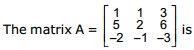Solution:
QUESTION: 16

If A = diag (2, –1, 3), B = diag (–1, 3, 2), then A2 B equal to

Solution:
QUESTION: 17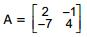and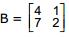then BTAT is

Solution:

A = {(2,-1) (-7,4)} B = {(4,1) (7,2)}
A’ = {(2,-7) (-1,4)} B’ = {(4,7) (1,2)}
B’A’ = {(1,0) (0,1)}
Therefore, identity matrix.

QUESTION: 18

If the matrix AB is a zero matrix, then

Solution:
QUESTION: 19

Which relation true for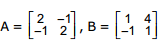Solution:
QUESTION: 20

If AB = A and BA = B, then B2  is equal to

Solution:

AB = A and BA = B
B2 = B.B
= (BA).B
= B.(AB)
= B.A
= B

QUESTION: 21

If A and B are symmetric matrices, then ABA is

Solution:

As A and B are symmetric matrices, therefore
A’ = A & B’ = B
So we have
(ABA)’ = A’ (AB)’= A’B’A’
= ABA ie symmetric matrix

QUESTION: 22

If A is a skew – symmetric matrix and n is an even positive integer, then An is

Solution:

A → skew symmetric matrix
n → positive integer
An → is symmetric if n is an odd integer and is skew symmetric if n is an even integer.

QUESTION: 23

If A is a non–singular matrix and AT denotes the transpose of A, then

Solution:

As we know that, |A| is not equal to zero
|A’| is also not equal to zero
So |A| + |A’| will not be equal to zero. Hence option d is correct.

QUESTION: 24

Which of the following is incorrect

Solution:
QUESTION: 25

If A is square matrix of order 3, then the true statement is (where l is unit matrix).

Solution:

If A is an n×n square matrix and n is odd, then
det(−A)=−det(A)
Proof:
det(−A)=(−1)n det(A) = −det(A)

QUESTION: 26

The transpose of a column matrix is a :

Solution:
QUESTION: 27

From the matrix equation AB = AC, we conclude B = C provided

Solution:

Let ∣A∣ is not equal to 0
∴A −1 exists.
Given AB=AC
Now, pre multiplying by A−1 on both sides. we get,
A−1(AB) = A−1(AC)
⇒ IB = IC
⇒ B = C
So, A is non singular

QUESTION: 28

Which of the following property of matrix multiplication is correct:

Solution:

Matrix multiplication is not commutative in general i.e., AB is not equal to BA.
Matrix multiplication is distributive over matrix addition i.e.,
(i) A(B+C)=AB+AC
(ii) (A+B)C=AB+AC, whenever both sides of equality are defined.
Matrix multiplication is associative i.e., (AB)C=A(BC), whenever both sides are defined.

QUESTION: 29

If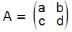satisfies the equation x2 – (a + d) x + k = 0, then

Solution:

Let λI = {(a,b) (c,d)}
{(λ,0) (0,λ)} - {(a,b) (c,d)}
= {(λ-a,0) (0,λ-d)} = 0
A =  {(λ-a,b) (c,λ-d)}
= λ2 - (a+d)λ + (ad+bc)
= A2 - (a+d)A + (ad+bc)
⇒ k = ad - bc

QUESTION: 30

Which of the following is a nilpotent matrix

Solution:

An n×n matrix A is called nilpotent if Ak=O, where O is the n×n zero matrix.
(a) The matrix A is nilpotent if and only if all the eigenvalues of A is zero.
(b) The matrix A is nilpotent if and only if An=O.
Therefore, option c gives zero matrix.